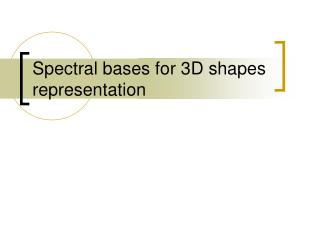# Spectral bases for 3D shapes representation - PowerPoint PPT PresentationDownload PresentationSpectral bases for 3D shapes representation

Spectral bases for 3D shapes representation
Download Presentation## Spectral bases for 3D shapes representation

- - - - - - - - - - - - - - - - - - - - - - - - - - - E N D - - - - - - - - - - - - - - - - - - - - - - - - - - -
##### Presentation Transcript

1. Spectral bases for 3D shapes representation

2. Spectral bases – reminder • Last week we spoke of Fourier analysis • 1D sine/cosine bases for signals:

3. Spectral bases – reminder • Last week we spoke of Fourier analysis • 2D bases for 2D signals (images)

4. How about 3D shapes? • Problem: general 3D shapes cannot be described as height functions Height function, regularly sampled above a 2D domain General 3D shapes

5. Irregular meshes • In graphics, shapes are mostly represented by triangle meshes

6. Irregular meshes • In graphics, shapes are mostly represented by triangle meshes • Geometry: Vertex coordinates (x1, y1, z1) (x2, y2, z2) . . . (xn, yn, zn) • Connectivity: List of triangles (i1, j1, k1) (i2, j2, k2) . . . (im, jm, km)

7. Irregular meshes • In graphics, shapes are mostly represented by triangle meshes • The connectivity (the graph) can be very efficiently encoded • About 2 bits per vertex only • The geometry (x,y,z) is heavy! • When stored naively, at least 12 bits per coordinate are needed, i.e. 36 bits per vertex

8. How to define efficient bases? • Extension of the 2D DCT basis to a general (irregular) mesh DCT ???

9. Basis shapes • We need a collection of basis functions • First basis functions will be very smooth, slowly-varying • Last basis functions will be high-frequency, oscillating • We will represent our shape (mesh geometry) as a linear combination of the basis functions • We will induce the basis functions from the mesh connectivity! • We assume that the connectivity is known to the decoder side (it is transferred first)

10. The Mesh Laplacian operator • Measures the local smoothness at each mesh vertex

11. Laplacian matrix • L is constructed based on the connectivity alone • So, if the decoder got the connectivity first, it can construct L

12. Spectral bases • L is a symmetric nn matrix • Perform spectral analysis of L! T 1 L 2 = n Basis vectors Frequencies, sorted in ascending order

13. How to represent our mesh geometry in the spectral basis? • X, Y, Z Rn. Decompose in the spectral basis:

14. How to represent our mesh geometry in the spectral basis? • Decompose the mesh geometry in the spectral basis: The first components are low-frequency The last components are high-frequency

15. The spectral basis • First functions are smooth and slow, last oscillate a lot chain connectivity horse connectivity 2nd basis function 10th basis function 100th basis function spectral basis of L = the DCT basis

16. Why this works? • Low-frequency basis vectors are smooth and slowly-varying: • The first basis vector: • It is the smoothest possible function (Laplacian is constant zero on it)

17. Why this works? • Low-frequency basis vectors are smooth and slowly-varying: • The first basis vector: • Lb1 = 0 because all the rows of L sum to zero

18. Why this works? • Low-frequency basis vectors are smooth and slowly-varying: • The following basis vectors: The frequency is small  the Laplacian operator gives small values  the shape of the function is smooth

19. Why this works? • High-frequency basis vectors are non-smooth, oscillating: The frequency is large  the Laplacian operator gives large values  the shape of the function is less smooth!

20. Geometry compression As in JPEG compression, drop the high-frequency components!

21. Geometry compression Increasing number of participating basis vectors. The less coefficients are dropped, the less shape information is lost

22. Technical algorithm • The encoding side: • Compute the spectral bases from mesh connectivity • Represent the shape geometry in the spectral basis and decide how many coeffs. to leave (K) • Store the connectivity and the K non-zero coefficients • The decoding side: • Compute the first K spectral bases from the connectivity • Combine them using the K received coefficients and get the shape

23. Challenges and trade-offs • Performing spectral decomposition of a large matrix (n>1000) is prohibitively expensive (O(n3)) • Today’s meshes come with 50,000 and more vertices • We don’t want the decompressor to work forever! • Solution: like in JPEG, work on small blocks:

24. Challenges and trade-offs • As in JPEG, blocking artifacts appear for low bit rates, because the compression is lossy (too much information is lost)

25. Further info • “Spectral Mesh Compression”, Zachi Karni and Craig Gotsman, ACM SIGGRAPH 2000 http://www.mpi-inf.mpg.de/~karni/publications/spectral.pdf • “3D Mesh compression using fixed spectral bases”, Z. Karni and C. Gotsman, Graphics Interface, 2001 http://www.mpi-inf.mpg.de/~karni/publications/fixed.pdf

26. See you next time